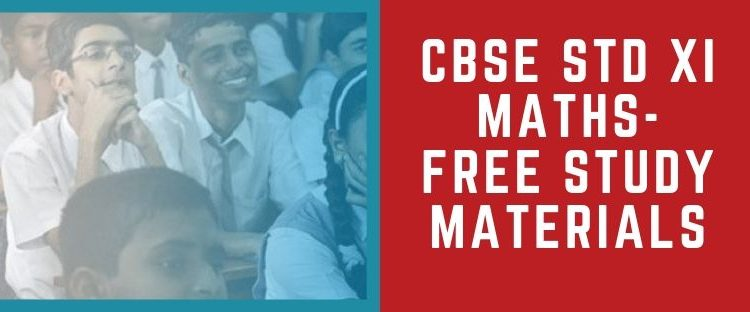CBSE Class 11 Maths Study Materials Free

All Chapter Study Notes, Solutions for Problems, Further referances and Video Lectures - All ChaptersUnderstanding the chapters of Class XI and gaining a good percentage for the CBSE XI Examination is of great significance as the syllabus of class 11 is included and is very crucial for the Class XII board examination. You can download complete learning assistance materials by experts from the links given below.

Mathematics being considered as one of the most important subject requires a lot of practice, dedication and reference to many study materials.  By referring different sources of study material, the students can get a clear idea of the chapter and also can understand many tricks to solve the sums in an easier effective manner. This knowledge would also help the students in their forthcoming education and entrance examinations.  Students failing to understand the concepts of class 11 may face lots of difficulties in class 12 and also in their future entrance examinations. So to help students understand each and every topic of class 11, we are here to provide study materials like Basics, Lecture Notes, Practice Papers, Reference Material etc.  They are shared for all the chapters of CBSE Class XI Mathematics.

Video links for each and every chapter of Class XI Mathematics are also provided to give the students a visual understanding on the topics covered.

Study Material of CBSE Class XI Mathematics:

 Chapter No. Chapter Name and Material Download Link 1 Sets 2 Relations And Functions 3 Trigonometric Functions 4 Principle Of Mathematical Induction 5 Complex Numbers And Quadratic Equations 6 Linear Inequalities 7 Permutations And Combinations 8 Binomial Theorem 9 Sequences And Series 10 Straight Lines 11 Conic Sections 12 Introduction To Three Dimensional Geometry 13 Limits And Derivatives 14 Mathematical Reasoning 15 Statistics

•ItsMeMoksha says:
•Saroj Meethal says: